﻿ canvas画布绘制动画基础 – 码云笔记

# canvas画布绘制动画基础

222℃

[隐藏]

## canvas绘制基础

### 创建Canvas

canvas是HTML中的一个新元素，本身和其它元素区别并不大，就是一个canvas标签，我们在浏览器中插入一个canvas标签，通常还会配一个ID，方便我们在JavaScript中获取canvas元素。

``<canvas id="myCanvas"></canvas>``

``<canvas id="canvas" style="border:1px solid #aaa;display:block;margin:50px auto;"></canvas>``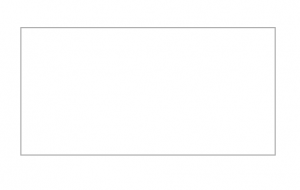``<canvas id="canvas" width="1024" height="768" style="border:1px solid #aaa;display:block;margin:50px auto;"></canvas>``

``````var canvas = document.getElementById("canvas");
var context = canvas.getContext("2d");//使用context进行绘制``````

``````window.onload = {
var canvas = getElementById("canvas");
var context = canvas.getContext("2d");
}``````

``````canvas.width = 1024;
canvas.height = 768;``````

``````<canvas id="canvas" style="border:1px solid #aaa;display:block;margin:50px auto;">

</canvas>``````

``````if(canvas.getContext("2d")){
var context = canvas.getContext("2d");
//使用context绘制
}else{
}``````

``````canvas.width= 1024；

canvas.height = 768;

canvas.getContext("2d");``````

### 绘制直线、多边形、七巧板

``````//draw a line
context.moveTo(100,100);
context.lineTo(700,700);

context.stroke();``````

``````context.moveTo(100,100);
context.lineTo(700,700);``````

``````window.onload = function() {
var canvas = document.getElementById("canvas");

canvas.width = 1024;
canvas,height = 768;

if(canvas.getContext("2d")){
var context = canvas.getContext("2d");
//使用context绘制
context.moveTo(100,100);
context.lineTo(700,700);
context.stroke();
}else{
}
}``````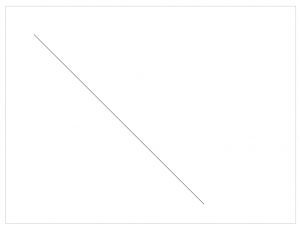``````context.lineWidth = 5;
context.strokeStyle = "#005588";``````

context.lineWidth是设置线条的宽度，context.strokeStyle描述线条样式，这个样式主要是指颜色，这个颜色可以使用CSS方法中任意方法类赋值，但是要放到一个字符串中,此时运行效果。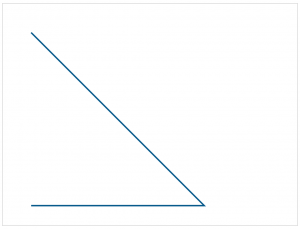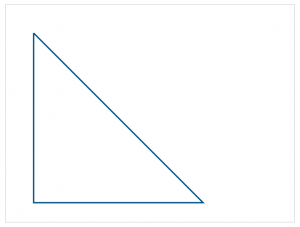``````context.fillStyle = "rgb(2,100,30)"
context.fill()````````````window.onload = function() {
var canvas = document.getElementById("canvas");

canvas.width = 1024;
canvas,height = 768;

if(canvas.getContext("2d")){
var context = canvas.getContext("2d");
//使用context绘制
context.moveTo(100,100);
context.lineTo(700,700);
context.lineTo(100,700);
context.lineTo(100,100);

context.fillStyle = "rgb(2,100,30)";
context.fill();

context.lineWidth = 5;
context.strokeStyle = "red";
context.stroke();
}else{
}
}``````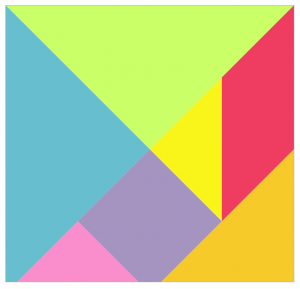``````var tangram = [
{p:[{x:0,y:0},{x:800,y:0},{x:400,y:400}],color:"#caff67"},
{p:[{x:0,y:0},{x:400,y:400},{x:0,y:800}],color:"#67becf"},
{p:[{x:800,y:0},{x:800,y:400},{x:600,y:600},{x:600,y:200}],color:"#ef3d61"},
{p:[{x:600,y:200},{x:600,y:600},{x:400,y:400}],color:"#f9f51a"},
{p:[{x:400,y:400},{x:600,y:600},{x:400,y:800},{x:200,y:600}],color:"#a594c0"},
{p:[{x:200,y:600},{x:400,y:800},{x:0,y:800}],color:"#fa8ecc"},
{p:[{x:800,y:400},{x:800,y:800},{x:400,y:800}],color:"#f6ca29"},
]``````

``````window.onload = function() {
var canvas = document.getElementById("canvas");

canvas.width = 800;
canvas,height = 800;
var context = canvas.getContext("2d");
//使用context绘制
for(var i = 0; i < tangram.length; i++){
draw(tangram[i],context);
}
}``````

``````function draw(piece, cxt) {
cxt.beginPath();
cxt.moveTo(piece.p.x, piece.p.y);
for(var i = 1; i < piece.p.length; i++){
cxt.lineTo(piece.p[i].x, piece.p[i].y);
}
cxt.closePath();
cxt.fillStyle = piece.color;
cxt.fill();

cxt.strokeStyle = "block";
cxt.lineWidth = 3;
cxt.stroke();
}``````

``````cxt.strokeStyle = "block";
cxt.lineWidth = 3;
cxt.stroke();``````

### 绘制弧和圆

``````context.arc(
startingAngle, endingAngle,
anticlockwise = false
)``````

centerx 表示圆的中心的 x 坐标

centery 表示圆的中心的 y 坐标

startingAngle 表示起始角，以弧度计。（弧的圆形的三点钟位置是 0 度）

endingAngle结束角，以弧度计

anticlockwise 可选。规定应该逆时针还是顺时针绘图。False = 顺时针，true = 逆时针。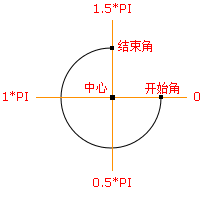• 中心：arc(100,75,50,0*Math.PI,1.5*Math.PI)
• 起始角：arc(100,75,50,0,1.5*Math.PI)
• 结束角：arc(100,75,50,0*Math.PI,1.5*Math.PI)

``````window.onload = function() {
var canvas = document.getElementById("canvas");

canvas.width = 800;
canvas.height = 800;
var context = canvas.getContext("2d");
//使用context绘制
context.lineWidth = 5;
context.strokStyle = "#005588";
context.arc(300,300,200,0,1.5*Math.PI);
context.stroke()
}````````context.arc(300, 300, 200, 0, 1.5*Math.PI, true);``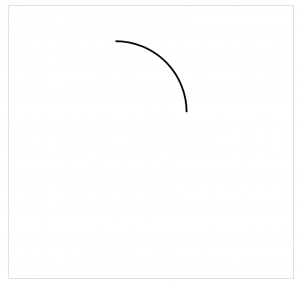``context.arc(300, 300, 200, 0, 0.5*Math.PI, true);````````window.onload = function() {
var canvas = document.getElementById("canvas");

canvas.width = 1024;
canvas,height = 768;
var context = canvas.getContext("2d");
//使用context绘制
context.lineWidth = 5;
context.strokStyle = "#005588";
//第一段
for(var i = 0; i < 10; i++){
context.beginPath();
context.arc(50 + i*100,60,40,0,2*Math.PI*(i+1)/10);
context.closePath();
context.stroke();
}
}````````````window.onload = function() {
var canvas = document.getElementById("canvas");

canvas.width = 1024;
canvas,height = 768;
var context = canvas.getContext("2d");
//使用context绘制
context.lineWidth = 5;
context.strokStyle = "#005588";
//第一段
for(var i = 0; i < 10; i++){
context.beginPath();
context.arc(50 + i*100,60,40,0,2*Math.PI*(i+1)/10);
context.closePath();
context.stroke();
}
//第二段
for(var i = 0; i < 10; i++){
context.beginPath();
context.arc(50 + i*100,180,40,0,2*Math.PI*(i+1)/10);
context.stroke();
}
}````````````window.onload = function() {
var canvas = document.getElementById("canvas");

canvas.width = 1024;
canvas,height = 768;
var context = canvas.getContext("2d");
//使用context绘制
context.lineWidth = 5;
context.strokStyle = "#005588";
//第一段
for(var i = 0; i < 10; i++){
context.beginPath();
context.arc(50 + i*100,60,40,0,2*Math.PI*(i+1)/10);
context.closePath();
context.stroke();
}
//第二段
for(var i = 0; i < 10; i++){
context.beginPath();
context.arc(50 + i*100,180,40,0,2*Math.PI*(i+1)/10);
context.stroke();
}
//第三段
for(var i = 0; i < 10; i++){
context.beginPath();
context.arc(50 + i*100,300,40,0,2*Math.PI*(i+1)/10, true);
context.closePath();
context.stroke();
}
}``````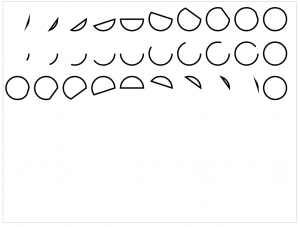``````context.fillStyle = "#005588";
for(var i = 0; i < 10; i++){
context.beginPath();
context.arc(50 + i*100,540,40,0,2*Math.PI*(i+1)/10);
context.closePath();
context.fill();
}
````````````for(var i = 0; i < 10; i++){
context.beginPath();
context.arc(50 + i*100,660,40,0,2*Math.PI*(i+1)/10);
context.fill();
}``````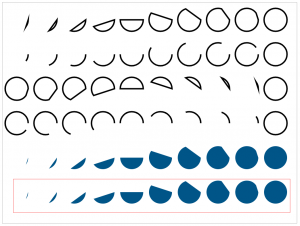## 结束语

canvas还为我们提供了更多的接口，在后续文章我们会结合案例穿插降解，根据今天所讲的内容我会针对性的带领大家实现一个炫酷的动态倒计时效果，敬请期待！

(2)个小伙伴在吐槽
1. 很详细的教程，楼主好人，哈哈
青菜萝卜干2018-12-04 07:27 回复